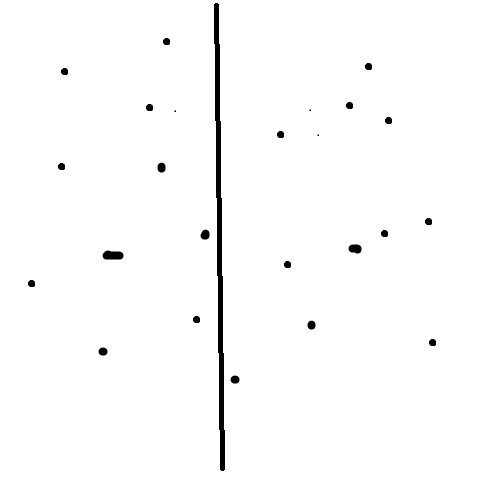最小覆盖双圆问题 | Kyons'Blog

## 前置知识:最小圆覆盖

给定平面一定数量的点,找一个最小的圆,将所有点包含进去.
然后,就不记得是哪位大佬提出了一种$O(n)$的解决方式,随机增量法.

1. 保证数据的随机性,我们使用$random\_shuffle$这个函数
2. 我们要接受一个定理:如果点$p$不在集合$S$的最小覆盖圆内,那么肯定在$p\cup S$的最小覆盖圆上.
3. 首先,假设我们已经求出了前$i$个点形成的最小覆盖圆
4. 如果$i+1$这个点不在$C_i$圆内,那么该$P_{i+1}$必定在$C_{i+1}$上
5. 那么我们以$P_{i+1}$为圆心,半径为$0$作为起始圆,找到第$j$个点,保证$dis\{i+1,j\}>$当前圆的半径.以这两点为圆$C_{i+1,j}$半径为$dis\{i+1,j\}$,圆心为$\frac{P_{i+1}+P_j}{2}$
6. 再寻找第三个点不在$C_{i+1,j}$,设为第$k$个点,那么以$i,j,k$三点的圆即可被认为是期望的最小覆盖圆$C_{i+1,j,k}$
7. 不断重复$3-6$步骤,即可得到答案.

体感就是$O(n^3)$的嘛,在数据不随机的情况下,会退化为$O(n^3)$,但在数据完全随机的情况下,预期期望复杂度为$O(n)$.

## 最小覆盖双圆问题//删除Valine核心代码库外链调用 //调用刚下载的本地文件以加速加载速度 //这里改为从本地加载